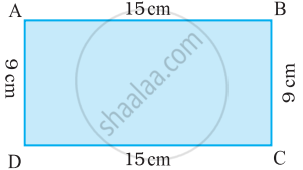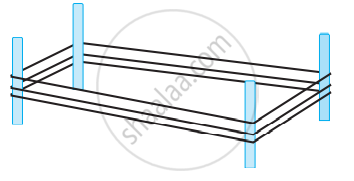# Perimeter of a Rectangle

## Formula

Perimeter of a rectangle = length + breadth + length + breadth

Perimeter of a rectangle = 2 × (length + breadth)

## Notes

### Perimeter of Rectangle:Perimeter of the rectangle = Sum of the lengths of its four sides.

= AB + BC + CD + DA

= AB + BC + AB + BC......(Opposite sides of Rectangle are equal)

= 2 × AB + 2 × BC

= 2 × (AB + BC)

= 2 × (15 cm + 9 cm)

= 2 × (24 cm)

= 48 cm

Hence, from the above example, we notice that

Perimeter of a rectangle = length + breadth + length + breadth

i.e. Perimeter of a rectangle = 2 × (length + breadth).

## Example

An athlete takes 10 rounds of a rectangular park, 50 m long and 25 m wide. Find the total distance covered by him.

Length of the rectangular park = 50 m
Breadth of the rectangular park = 25 m
Total distance covered by the athlete in one round will be the perimeter of the park.
Now, perimeter of the rectangular park = 2 × (length + breadth)

= 2 × (50 m + 25 m)

= 2 × 75 m

= 150 m

So, the distance covered by the athlete in one round is 150 m.

Therefore, distance covered in 10 rounds = 10 × 150 m = 1500m

The total distance covered by the athlete is 1500 m.

## Example

A farmer has a rectangular field of length and breadth 240 m and 180 m respectively. He wants to fence it with 3 rounds of rope as shown in the figure. What is the total length of rope he must use?The farmer has to cover three times the perimeter of that field.

Therefore, the total length of rope required is thrice its perimeter.

Perimeter of the field = 2 × (length + breadth)

= 2 × ( 240 m + 180 m)

= 2 × 420 m

= 840 m

Total length of rope required = 3 × 840 m = 2520 m.

## Example

Find the cost of fencing a rectangular park of length 250 m and breadth 175 m at the rate of Rs. 12 per metre.

Length of the rectangular park = 250 m

Breadth of the rectangular park = 175 m

Perimeter of the rectangle = 2 × (length + breadth)

= 2 × (250 m + 175 m)

= 2 × (425 m)

= 850 m

Cost of fencing 1m of park = Rs. 12

Therefore, the total cost of fencing the park = Rs. 12 × 850 = Rs. 10200

If you would like to contribute notes or other learning material, please submit them using the button below.

### Shaalaa.com

Perimeter Of A Rectangle [00:06:55]
S
0%